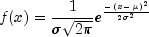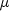JMSLTM Numerical Library 7.2.0
com.imsl.stat

## Class NormalDistribution

• All Implemented Interfaces:
Distribution, ProbabilityDistribution, Serializable

```public class NormalDistribution
extends Object
implements ProbabilityDistribution, Serializable```
Evaluates the normal (Gaussian) probability density for a given set of data.

`NormalDistribution` evaluates the normal probability density of a given set of data, `xData`. If parameters are not supplied, the `eval` method fits the normal probability density function to the data by first calculating the mean and standard deviation of `xData`. The normal probability density function is defined as:whereandare the mean and standard deviation.

The `DataMining` package class `NaiveBayesClassifier` uses `NormalDistribution` as the default method to train continuous data.

Example, Serialized Form
• ### Constructor Summary

Constructors
Constructor and Description
`NormalDistribution()`
• ### Method Summary

Methods
Modifier and Type Method and Description
`double[]` `eval(double[] xData)`
Fits a normal (Gaussian) probability distribution to `xData` and returns the probability density at each value.
`double[]` ```eval(double[] xData, Object[] parameters)```
Evaluates a normal (Gaussian) probability distribution with the given parameters at each point in `xData` and returns the probability density at each value.
`double` ```eval(double xData, Object[] parameters)```
Evaluates a normal (Gaussian) probability density at a given point `xData`.
`double` `getMean()`
Returns the population mean of `xData`.
`Object[]` `getParameters()`
Returns the current parameters of the normal probability density function.
`double` `getStandardDeviation()`
Returns the population standard deviation.
• ### Methods inherited from class java.lang.Object

`clone, equals, finalize, getClass, hashCode, notify, notifyAll, toString, wait, wait, wait`
• ### Constructor Detail

• #### NormalDistribution

`public NormalDistribution()`
• ### Method Detail

• #### eval

`public double[] eval(double[] xData)`
Fits a normal (Gaussian) probability distribution to `xData` and returns the probability density at each value.
Specified by:
`eval` in interface `Distribution`
Parameters:
`xData` - a `double` array representing the points at which the normal probability distribution function is to be evaluated
Returns:
a `double` array representing the normal probability density at each value in `xData`
• #### eval

```public double[] eval(double[] xData,
Object[] parameters)```
Evaluates a normal (Gaussian) probability distribution with the given parameters at each point in `xData` and returns the probability density at each value.
Specified by:
`eval` in interface `ProbabilityDistribution`
Parameters:
`xData` - a `double` array representing the points at which the normal probability distribution function is to be evaluated
`parameters` - an `Object` array representing the parameters used to evaluate the normal probability density function, see method `getParameters`
Returns:
a `double` array representing the normal probability density of each value in `xData`
• #### eval

```public double eval(double xData,
Object[] parameters)```
Evaluates a normal (Gaussian) probability density at a given point `xData`.
Specified by:
`eval` in interface `ProbabilityDistribution`
Parameters:
`xData` - a `double` containing the point at which the normal probability density function is to be evaluated
`parameters` - an `Object` array representing the parameters used to evaluate the normal probability density, see method `getParameters`
Returns:
a `double` representing the normal probability density at `xData`
• #### getMean

`public double getMean()`
Returns the population mean of `xData`.
Returns:
a `double` representing the population mean of `xData`
• #### getParameters

`public Object[] getParameters()`
Returns the current parameters of the normal probability density function.
Specified by:
`getParameters` in interface `ProbabilityDistribution`
Returns:
an `Object` array containing the parameters resulting from the last invocation of the (`Distribution`) `eval` method with the following signature, `double[] eval(double[] xData)`. This `Object` array can be used as input to the `eval` methods that require an `Object` array of distribution parameters as input.
• #### getStandardDeviation

`public double getStandardDeviation()`
Returns the population standard deviation.
Returns:
a `double` representing the population standard devation of `xData`
JMSLTM Numerical Library 7.2.0